# Simple and Efficient Double-Talk-Detector for Acoustic Echo Cancellation

Simple and Efficient Double-Talk-Detector for Acoustic Echo Cancellation
Electronics Department, Faculty of Technology, University of M’sila, M'sila 28000, Algeria

LASS, Laboratory of Analysis of Signals and Systems, M'sila 28000, Algeria

Corresponding Author Email:
Page:
585-592
|
DOI:
https://doi.org/10.18280/ts.370406
10 May 2020
|
Accepted:
28 July 2020
|
Published:
10 October 2020
| Citation

OPEN ACCESS

Abstract:

Acoustic Echo Cancellation (AEC) is a topic that has received a great interest in recent years. However, a significant challenge remains with the problem of double-talk especially when the adaptive filter has a fast convergence rate. In this case, the double-talk detector (DTD) must reply in early stage and halt updating of the adaptive filter in order to avoid filter coefficients divergence. Indeed, a complex and inappropriate DTD can seriously affect the convergence rate of the adaptive filter and global performances of the AEC system. In this paper, an implementation of a simple and efficient DTD based on a recursive estimation of the decision variable which is resulting from the level comparison between far-end and microphone signals is proposed. The presented algorithm is then compared with the normalized cross-correlation (NCC) method which is taken as a reference in this work. In the simulation tests, the recursive least squares (RLS) algorithm is used to update the adaptive filter coefficients. The speech signals used in the tests are taken from the TIMIT database.

Keywords:

AEC, DTD, RLS, Geigel algorithm, NCC, recursive estimation

1. Introduction

In the last years, research on acoustic echo cancellation (AEC) has become very popular, particularly with the large use of teleconference and hands-free communication systems [1-4]. The first echo cancellers researches appear for the first time in 1967. Afterwards, many developments have been attempted in order to increase performances and reduce computational complexities [5-9]. AEC systems essentially based on adaptive filters, can be classified into two main categories, least mean squares (LMS) algorithms and recursive least squares (RLS) algorithms. Compared to LMS, RLS proved its effectiveness in terms of convergence rate at the cost of increasing computational complexity [10-14]. The performance of RLS algorithms can be controlled using two parameters: forgetting factor λ and regularization term Δ .

One of the main components of AEC systems based on adaptive filters is the double-talk-detector (DTD). The properties of this device play an important role in the global system performance. It is used to halt updating of the adaptive filter during the presence of near-end speech which can seriously affect the filter adaptation and causes its divergence. However, a reliable DTD must distinguish between a double-talk (DT) situation, and any change occurred in the echo-path. In the literature, many solutions have been proposed in order to improve the performances of DTDs [16-20]. These solutions are based on different mathematical formulations and using different techniques. The purpose has been often the improvement of the performances without attributing importance to the computational complexity of the DTD. Many other methods based on combined adaptive filtering have been attracting considerable attention in the field of acoustic echo cancellation. These methods are based on another strategy of echo cancellation without DTD, which retains the advantages of both fast convergence rate and small steady-state misalignment. Furthermore, they suffer from the same problems encountered in this field and consume more computing time [21, 22].

In this work, we are interested to solve the problem produced by the acoustic echo in case of double-talk periods based on DTD. We present here a new algorithm based on a recursive estimation of the decision variable resulting from the far-end signal to the microphone signal ratio. The main properties of this proposed method are simplicity, efficiency, and low computational complexity. To demonstrate these performances, a comparison with the normalized cross-correlation (NCC) is presented. NCC method is taken in this work as a reference because of its high efficiency and robustness .

The remainder of the paper is organized as follows: Section 2 presents the general concept of acoustic echo canceller and the theoretical background of RLS algorithms. Section 3 details the different algorithms used in this study. The simulation results are given in Section 4. Finally, the section 5 concludes the paper.

2. Acoustic Echo Cancellation

2.1 General concept

The purpose of acoustic echo canceller is to remove as much as possible the echo due to the acoustic coupling between loudspeaker and microphone in a hands-free communication system. In practice, the echo signal y(n) is the result of the passage of far-end signal x(n) through the echo-path. It is picked up with the near-end signal v(n) by the microphone. The microphone signal denoted d(n) is derived as follows:

\begin{aligned} d(n) &=y(n)+v(n)+b(n) \\ &=\mathbf{h}^{T} \mathbf{x}_{L}(n)+v(n)+b(n) \end{aligned}      (1)

where:

hT=[h0 h1…hL-1]: is the impulse response of the echo-path with a tap-length L.

$x_{L}^{T}(n)=[x(n) x(n-1) \ldots x(n-L+1)]$: is the vector of L past samples of the far-end signal.

b(n):is the noise signal.

The echo cancellation is carried out by subtracting a replica of echo from the microphone signal d(n). The estimated echo is a linear combination of the far-end signal x(n) with the adaptive filter:

$\hat{y}(n)=\mathbf{w}^T \mathbf{x}_{L}(n)$    (2)

where: $\mathbf{w}^{T}(n)=\left[w_{0}(n) w_{0}(n) \ldots w_{L-1}(n)\right]$

Thereby, we get the error signal by:

$e(n)=d(n)-\hat{y}(n)$       (3)

Ideally, the error signal is almost equal to zero when the near-end speech is not present. With the assumption of no noisy environment and no changes in the echo-path, the echo cancellation remains a simple problem of identification. However, in DT periods or changes in the echo-path, the identification becomes more complex. In order to solve partially this problem, a DTD is recommended.

In this work, RLS which allows updating of the filter coefficients is used to validate the proposed DTD [10, 13].

The adaptive filter minimizes, at time n, the weighted least squares error:

\begin{aligned} J(n) &=\sum_{i=0}^{n} \lambda^{n-i}[e(i)]^{2} \\ &=\sum_{i=0}^{n} \lambda^{n-i}\left[d(i)-\mathbf{w}_{n}^{T} \mathbf{x}(i)\right]^{2} \end{aligned}    (4)

where: 0<λ≤1, it is an exponential weighting (forgetting) factor.

We present in Table 1 a summary of this algorithm.

Table 1. RLS algorithm summary

 Parameters $L=$filter order $\lambda=$ forgetting factor $\delta=$ regularization paramerter Initialization: $w_{0}=0$ $P(0)=\delta^{-1} I$ Computation: $z(n)=P(n-1) x(n)$ $k(n)=\frac{1}{\lambda+x^{T}(n) z(n)} z(n)$ $e(n)=d(n)-w_{n-1}^{T} x(n)$ $P(n)=\frac{1}{\lambda}\left[P(n-1)-k(n) z^{T}(n)\right]$ $w_{n}=w_{n-1}+e(n) k(n)$
3. Double-Talk Detection

One of the main problems of AEC systems based on adaptive filtering is the degradation caused by the near-end signal in DT periods. In this case, updating of the adaptive filter coefficients must be stopped by DTD to avoid divergence.

Several methods have been proposed to design DTDs, i.e. Geigel algorithm  based on the level comparison between far-end and microphone signals, coherence , NCC algorithms between far-end and error signals , between far-end and microphone signals  and between microphone and error signals . Many other recent methods are used, i.e. method based on the soft decision  and method based on the Stockwell transform .

3.1 Geigel algorithm

Geigel algorithm is a conventional method that is widely used for its simplicity and its easy implementation. It is usually limited to network echo application, where the echo level is typically 6 dB below the level of far-end speech. It performs an amplitude level comparison between the maximum of LG observations of signal x(n) and the microphone signal d(n). Parameter LG is a constant that determines the number of past samples of the far-end signal used by the DTD. The decision variable is then defined:

$\xi_{G}(n)=\frac{\max \left\{|x(n)|, \mid x\left(n-1|, \ldots \ldots,| x\left(n-L_{G}+1\right) \mid\right\}\right.}{|d(n)|}$     (5)

ξG(n) is made by comparing with a suitable threshold level TG where its value depends on the context use of the echo canceller. In Figure 1 we represent an echo canceller based on the Geigel DTD.

## 1.png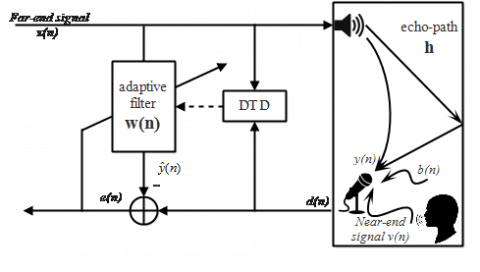Figure 1. Echo canceller based on the Geigel DTD

In the literature, the conventional Geigel algorithm is always shown as a basic method with poor performance. However, the NCC method has good properties and will be taken as a reference in this study.

3.2 Normalized cross-correlation algorithm

The first method based on the cross-correlation between the far-end signal and the error signal is proposed by Ye and Wu . Some approximate versions of NCC are appeared in different articles [23, 27, 30]. Each method differs from the others in the DTD input signals. The method which depends on the cross-correlation between the microphone signal and the error signal  is used in the comparative study with our proposed algorithm. We note that the performance of the proposed method  is exactly similar to the best-known cross-correlation based on double-talk-detector .

A statistical decision ξNCC of the NCC method is given by :

$\xi_{N C C}=1-\frac{\hat{r}_{e d}}{\hat{\sigma}_{d}^{2}}$     (6)

where:

$\hat{r}_{e d}=E\{e(n) d(n)\}$, it is the cross-correlation between e(n) and d(n);

$\hat{\sigma}_{d}^{2}$, it is the variance of d(n).

It should be noted that the proposed method  has good performances compared to several other methods including the conventional Geigel algorithm. For evaluation, we propose to compare this interesting method with our proposed algorithm.

3.3 Proposed algorithm

In this section, we present a simple method of double-talk-detection based on a recursive estimation of the decision variable which is resulting from the far-end signal to the microphone signal ratio. The evaluation of the decision variable is according to the exponential recursive weighting algorithm .

We note this decision variable as:

$\xi_{E s}=\frac{\hat{\mathrm{m}}_{x}(n)}{\hat{d}(n)}$     (7)

with:

$\widehat{m}_{x}(n)=\alpha \hat{m}_{x}(n-1)+(1-\alpha)|x(n)|$    (8)

$\hat{d}(n)=\alpha|\hat{d}(n-1)|+(1-\alpha)|d(n)|$      (9)

where: α = 0.99

$\hat{m}_{x}(n)$ and $\hat{d}(n)$ represent respectively the estimations of the last samples of far-end and microphone speech signals.

x(n) and d(n) are respectively the current samples of far-end and microphone speech signals.

α, it is the exponential weighting factor. Smaller values of α, involve better tracking capability but to the detriment of estimation accuracy. For slowly time varying signals, α is usually chosen ≈ 1 .

The decision variable is obtained by comparison with a suitable threshold level T evolving adaptively in time. The binary decision is calculated as follows:

If DT detected, the binary decision=1.

If DT not detected, the binary decision=0.

4. Simulation Results

A measured acoustic impulse response of a car cockpit is used in this simulation (Figure 2). It is sampled at 16 kHz with a tap-length of L=512 [32, 33]. Near-end and far-end signals of three scenarios (Sc1, Sc2, Sc3) represented in Figure 3, are provided from the TIMIT database . In the first step tests, scenario Sc1 is used to compare the properties of the different methods.

## 2.png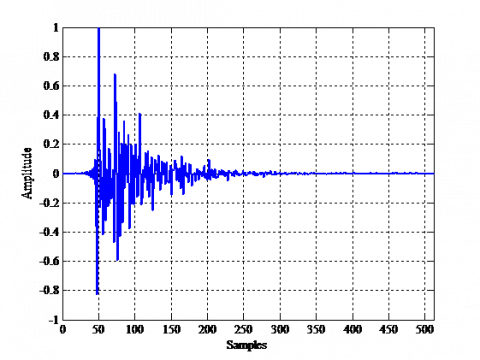Figure 2. Car cockpit impulse response

## 3.png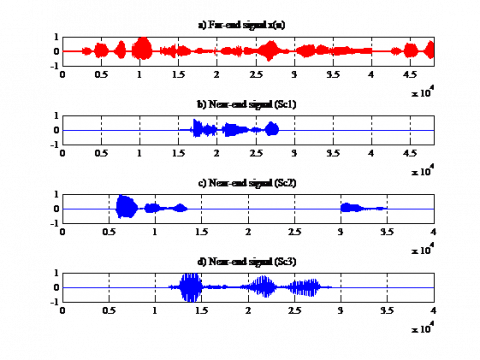Figure 3. Speech signals: (a). far-end signal. (b). near-end signal (Sc1). (c). near-end signal (Sc2). (d) near-end signal (Sc3)

The various parameters (λ and Δ) of the RLS algorithm are determined empirically after several tests where it is necessary to make a compromise (λ= 0.9995 and Δ=0.05).

To evaluate the performances of the different methods, three criteria are used: misalignment, echo-return loss enhancement (ERLE) and the probability of miss detection (Pm) which are given as follows [29, 35]:

Misalignment $(d B)=10 \log _{10}\left[\frac{\|\mathbf{w}(n)-\mathbf{h}\|^{2}}{\|\mathbf{h}\|^{2}}\right]$      (10)

$E R L E(d B)=10 \log _{10}\left\{\frac{E\left[|d(n)|^{2}\right]}{E\left[|e(n)|^{2}\right]}\right\}$     (11)

$P_{m}=1-\frac{\sum_{n=1}^{N} \bar{x}(n) \bar{v}(n) \phi(n)}{\sum_{n=1}^{N} \bar{x}(n) \bar{v}(n)}$      (12)

Pm, it is defined as the probability of detection failure when DT is present.

$\bar{x}(n)$ is the voice activity detection of far-end signal $x(n)$. $\bar{v}(n)$ is the voice activity detection of near-end signal $v(n)$. $\phi(n)$ is the binary decision of the DTD method and $\mathrm{N}$ is the length of x(n).

In Figure 4, misalignments are represented. The obtained results demonstrate the superiority of the proposed method compared to the two others. Slow convergence of the Geigel algorithm is due to its great number of false alarms. However, the divergence of NCC indicates its high sensitivity to the presence of DT situations. The proposed method presents a compromise of good convergence and high stability during the DT period.

## 4.png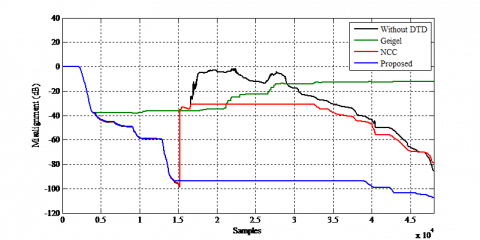Figure 4. Misalignments evaluation with scenario Sc1

Criterion of ERLE is considered as one of the most used in performance measurements of AEC systems. We note that recommendation G.131 of the International Telecommunications Union (ITU) requires attenuation of more than 40 dB in the absence of double-talk .

The obtained results presented in Figure 5 confirm the superiority of the proposed method with an echo attenuation of more than 50 dB in period A and more than 100 dB in period C.

We show in Table 2 an evaluation of ERLE values obtained from periods (A, B, C) with scenario Sc1.

## 5.png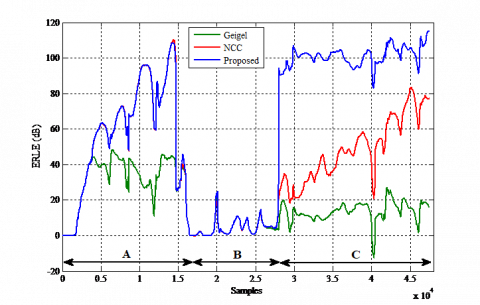Figure 5. ERLE evaluation with scenario Sc1

All the previous simulations are performed with supposing that the echo signal is not affected by the background noise.

In the current section, we use an independent white Gaussian noise added to the echo signal y(n) with different signal to noise ratio (SNR) defined as:

$S N R(d B)=10 \log _{10}\left\{\frac{E\left[|y(n)|^{2}\right]}{E\left[|b(n)|^{2}\right]}\right\}$       (13)

In Table 3, we show an evaluation of ERLE values obtained for the three methods in a noisy environment with scenario Sc1. According to the Figure 6, we observe clearly the superiority of the ERLE average obtained by the proposed algorithm.

## 6.png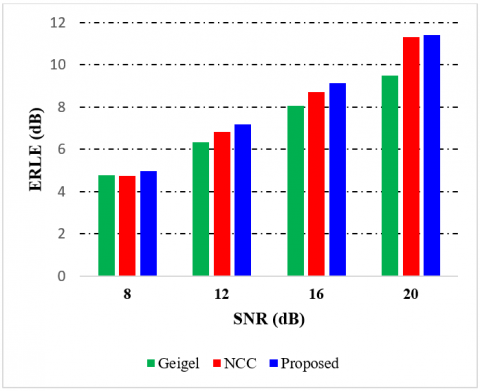Figure 6. Evolution of ERLE average in a noisy environment with scenario Sc1

The misalignments comparison in a noisy environment is given in Figure 7. We observe that in the majority of cases, the proposed algorithm proves the best performance in terms of convergence and small steady-state misalignment.

In order to confirm the efficiency of the proposed algorithm, we will proceed to test the scenarios Sc2 and Sc3 where the double-talk periods are omnipresent. We present in Table 4 the parameter values for scenarios (Sc2, Sc3), parameter values of ERLE obtained in a noisy environment with scenario Sc1 and Sc2. We observe that the proposed algorithm demonstrates the best ERLE average with an SNR varying between 8 dB and 20 dB.

To simulate an abrupt change in the echo-path, we have increased the gain of the acoustic channel by 4 at sample 24000. The Figure 8 demonstrates that the proposed algorithm shows the best performance compared to Geigel and NCC algorithms in terms of convergence, small steady-state misalignment and tracking capability. Indeed, the sensitivity and stability of its decision variable may have countered the abrupt change efficiently. Furthermore, the NCC method is highly sensitive as well as changes in the near-end speech. However, it appears that it is unable in this case to deal efficiently with the change in the echo-path.

Table 2. Parameter values of ERLE obtained from the three periods with scenario Sc1

 Period Geigel NCC Proposed Min Max Mean Min Max Mean Min Max Mean A -16.75 48.44 29.98 -0.19 110.30 53.82 -0.16 108.49 53.88 B -0.24 23.70 4.33 -0.27 22.31 4.50 -0.24 23.70 4.68 C -12.63 27.33 13.59 18.35 83.65 48.54 51.29 115.42 100.94

Table 3. Parameter values of ERLE in a noisy environment with scenario Sc1

 SNR (dB) Geigel NCC Proposed Min Max Mean Min Max Mean Min Max Mean 8 -0.5 30.53 4.77 -5.91 40.08 4.73 -3.98 40.10 4.97 12 -0.47 60.67 6.33 -2.39 40.10 6.82 -1.50 40.08 7.17 16 -0.51 30.57 8.05 -4.37 40.23 8.70 -0.86 40.04 9.13 20 -0.92 30.69 9.48 -0.54 40.05 11.31 -0.42 40.04 11.42

Table 4. Parameter values of ERLE with different SNR for the two scenarios Sc2 and Sc3

 SNR (dB) Sc Geigel NCC Proposed Min Max Mean Min Max Mean Min Max Mean 8 Sc2 -1.63 30.50 4.81 -1.63 40.07 4.88 -2.02 40.05 5.24 Sc3 -0.94 30.50 4.55 -1.29 39.99 4.56 -3.33 40.10 4.80 12 Sc2 -1.57 30.57 6.40 -2.70 40.04 6.81 -2.02 40.03 7.15 Sc3 -1.33 30.28 5.91 -1.78 40.09 6.06 -1.25 40.07 6.63 16 Sc2 -1.74 30.61 7.99 -2.44 40.13 8.65 -1.91 40.01 9.19 Sc3 -1.41 30.70 7.17 -1.41 40.08 7.73 -1.59 40.02 8.42 20 Sc2 -1.8 30.59 9.26 -1.80 40.10 10.93 -1.65 40.05 11.15 Sc3 -1.19 30.70 8.32 -1.64 40.10 9.72 -1.31 40.09 10.22

## 7a.png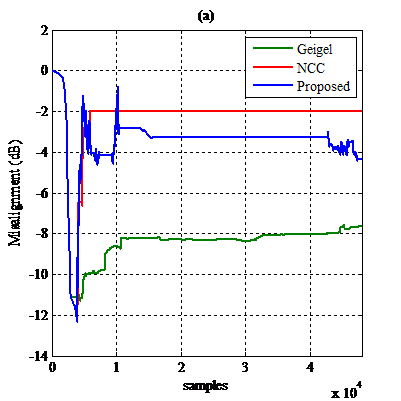## 7b.png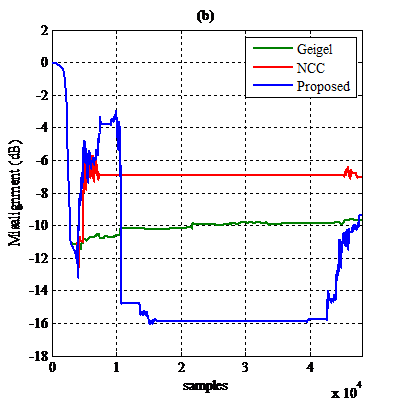## 7c.png## 7d.png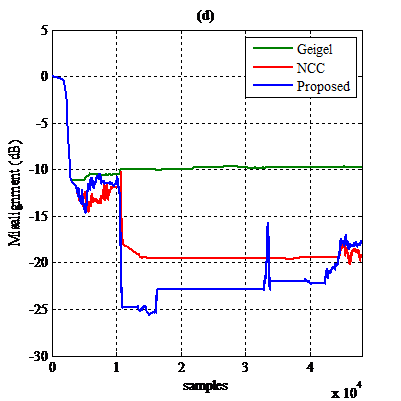Figure 7. Misalignments evaluation in a noisy environment with scenarion Sc1. (a) SNR= 8 dB. (b) SNR= 12 dB. (c) SNR= 16 dB. (d) SNR= 20 dB

## 8.png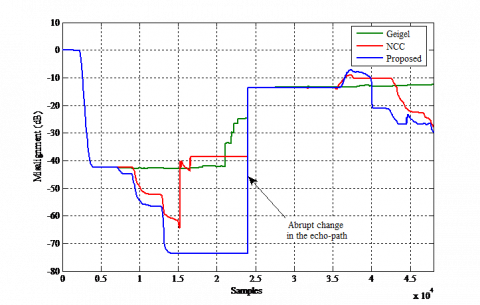Figure 8. Misalignments evaluation with a change in the echo-path

To avoid the empiric choice of the threshold, an adaptive threshold T evolving with time is proposed and calculated according to the exponential recursive weighting algorithm.

$T(n)=\beta T(n-1)+(1-\beta) \xi_{E s}(n)$      (14)

where: β= 0.99

The detailed steps of the adaptive threshold algorithm are given in Figure 9.

## 9.png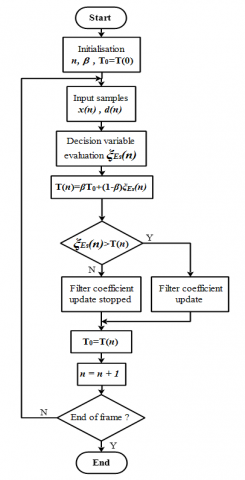In Figure 10, we present the misalignments obtained with the proposed algorithm using adaptive and different fixed values of the threshold. Noting that T=0.78 is the best value used in previous simulations.

## 10.png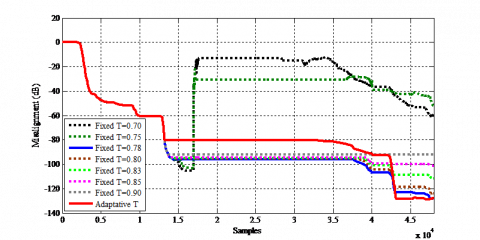Figure 10. Misalignments obtained with adaptive and different fixed values of the threshold T for the proposed method

Objective performance evaluation based on the probability of missing detection Pm is presented in Figure 11. It is calculated as a function of NFR (Near-Far-end-Ratio) values varying between -18 dB and 10 dB. The used threshold for each method is chosen to give a probability of false detection Pf =0.4 which is defined as the probability of declaring detection when DT does not exist. It is calculated without a near-end signal as:

$P_{f}=\frac{1}{N} \sum_{n=1}^{N} \bar{x}(n) \phi(n)$      (15)

This evaluation demonstrates that the proposed algorithm is better than Geigel and NCC in terms of the probability of missing detection when NFR varies more than -10 dB.

## 11.png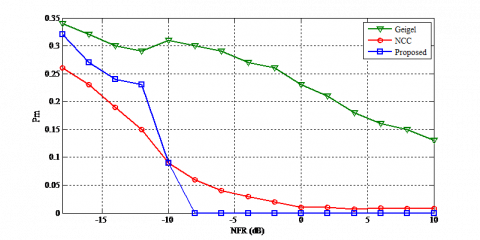Figure 11. Probability of missing detection

Table 5. Computational complexity per iteration

 Algorithm Add Sub Mul Div Comparisons Geigel 0 0 0 1 L-1 NCC 2 1 6 1 0 Proposed 2 2 4 1 0

Finally, to evaluate the computational complexity, we summarize in Table 5 the number of operations per iteration used in each algorithm. Noting that the complexity of the Geigel algorithm depends directly on the tap-length LG of the window used to calculate the max of x(n) samples. On the contrary, the proposed algorithm and NCC are independent regardless of this parameter. Furthermore, the proposed algorithm remains faster than NCC and its decision variable is computed with only 7 operations (4 multiplications, 2 additions and 1 division) per iteration.

5. Conclusion

In this paper, a simple and efficient method for double-talk-detection is proposed. To confirm its performance, an evaluation has been performed using three criteria: misalignment, ERLE, and the probability of miss detection. The proposed method has demonstrated the best performance compared to Geigel and NCC methods. Nice properties have been obtained in terms of convergence, small steady-state misalignment, high ERLE, and robustness against the additive white noise and abrupt change in the echo-path. The proposed algorithm has demonstrated a high ability to halt in early stage, updating of the adaptive filter coefficients during the double-talk periods. It has presented regardless to Geigel and NCC, an improvement in terms of minimizing the number of missing detections when NFR varied more than -10 dB. To support efficiency of our method and to avoid preliminarily the empiric choice of the threshold, an adaptive threshold T evolving with time has been proposed. Furthermore, our algorithm can be considered more efficient for optimizing computation time with fewer arithmetic operations. Indeed, we consider that it is significantly simpler and has the capability to outperform the NCC method used as a reference in this work. We may surmise from the obtained results that the proposed algorithm is suitable for efficient echo cancellers in communication systems.

Future work will investigate the proposed method in comparison to other recent methods.

References

 Benesty, J., Gänsler, T., Morgan, D.R., Sondhi, M.M., Gay, S.L. (2001). Advances in network and acoustic echo cancellation. Digital Signal Processing. Springer, Berlin, Heidelberg. https://doi.org/10.1007/978-3-662-04437-7_5

 Hänsler, E., Schmidt, G. (Eds.). (2006). Topics in acoustic echo and noise control: selected methods for the cancellation of acoustical echoes, the reduction of background noise, and speech processing. Springer Science & Business Media. http://dx.doi.org/10.1007/3-540-33213-8

 Benesty, J., Paleologu, C., Gänsler, T., Ciochină, S. (2011). A perspective on stereophonic acoustic echo cancellation. Springer Science & Business Media, 4. http://dx.doi.org/10.1007/978-3-642-22574-1

 Halimeh, M.M., Kellermann, W. (2020). Efficient multichannel nonlinear acoustic echo cancellation based on a cooperative strategy. In ICASSP 2020-2020 IEEE International Conference on Acoustics, Speech and Signal Processing (ICASSP), pp. 461-465. https://doi.org/10.1109/ICASSP40776.2020.9054541

 Sondhi, M.M. (1967). An adaptive echo canceller. Bell System Technical Journal, 46(3): 497-511. http://dx.doi.org/10.1002/j.1538-7305.1967.tb04231.x

 Sankar, S., Kar, A., Burra, S., Swamy, M.N.S., Mladenovic, V. (2020). Nonlinear acoustic echo cancellation with kernelized adaptive filters. Applied Acoustics, 166: 107329. https://doi.org/10.1016/j.apacoust.2020.107329

 Sankar, S., Kar, A., Burra, S., Swamy, M.N.S., Mladenovic, V. (2000). Step-size control for echo cancellation filters-an overview. Signal Processing, 80(9): 697-1719. https://doi.org/10.1016/S0165-1684(00)00082-7

 Gilloire, A., Vetterli, M. (1992). Adaptive filtering in sub-bands with critical sampling: Analysis, experiments, and application to acoustic echo cancellation. IEEE Transactions on Signal Processing, 40(8): 1862-1875. https://doi.org/10.1109/78.149989

 Benesty, J., Morgan, D.R., Sondhi, M.M. (1998). A better understanding and an improved solution to the specific problems of stereophonic acoustic echo cancellation. IEEE Transactions on Speech and Audio Processing, 6(2): 156-165. https://doi.org/10.1109/89.661474

 Haykin, S.S. (1995). Adaptive Filter Theory. ISBN: 9780132671453

 Hayes, M.H. (1996). Statistical Digital Signal Processing and Modeling. John Wiley& Sons, Inc.

 Husoy, J.H., Abadi, M.S.E. (2004). A comparative study of some simplified RLS-type algorithms. In First International Symposium on Control, Communications and Signal Processing, pp. 705-708. https://doi.org/10.1109/ISCCSP.2004.1296509

 Chergui, L., Bouguezel, S. (2019). A new post-whitening transform domain LMS algorithm. Traitement du Signal, 36(3): 245-252. https://doi.org/10.18280/ts.360307

 Yang, B.H. (2020). An adaptive filtering algorithm for non-Gaussian signals in alpha-stable distribution. Traitement du Signal, 37(1): 69-75. https://doi.org/10.18280/ts.370109

 Elisei-Iliescu, C., Paleologu, C. (2017). Recursive least-squares algorithms for echo cancellation-an overview and open issues. The Sixteenth International Conference on Networks.

 Benziane, M., Bouamar, M., Makdir, M. (2018). Double-talk detection based on enhanced Geigel algorithm for acoustic echo cancellation. In 2018 6th International Conference on Control Engineering & Information Technology (CEIT), pp. 1-5. https://doi.org/10.1109/CEIT.2018.8751867

 Gänsler, T., Benesty, J., Gay, S.L. (2000). Double talk detection schemes for acoustic echo cancellation, acoustic signal processing for telecommunication. Springer Science Business Media, pp. 81-95. http://dx.doi.org/10.1007/978-1-4419-8644-3_5

 Lee, K.H., Chang, J.H., Kim, N.S., Kang, S., Kim, Y. (2010). Frequency-domain double-talk detection based on the Gaussian mixture model. IEEE Signal Processing Letters, 17(5): 453-456.https://doi.org/10.1109/LSP.2010.2043891

 Jenq, J.C., Hsieh, S.F. (2001). Acoustic echo cancellation using iterative-maximal-length correlation and double-talk detection. IEEE Transactions on Speech and Audio Processing, 9(8): 932-942. https://doi.org/10.1109/89.966096

 Sugiyama, A., Berclaz, J., Sato, M. (2005). Noise-robust double-talk detection based on normalized cross correlation and a noise offset. IEEE International Conference on Acoustics, Speech, and Signal Processing, Philadelphia, PA, 3: iii/153-iii/156. https://doi.org/10.1109/ICASSP.2005.1415669

 Huang, F., Zhang, J., Zhang, S. (2015). Combined-step-size affine projection sign algorithm for robust adaptive filtering in impulsive interference environments. IEEE Transactions on Circuits and Systems II: Express Briefs, 63(5): 493-497. http://dx.doi.org/10.1109/TCSII.2015.2505067

 Chien, Y.R., Li-You, J. (2018). Convex combined adaptive filtering algorithm for acoustic echo cancellation in hostile environments. IEEE Access, 6: 16138-16148. http://dx.doi.org/10.1109/ACCESS.2018.2804298

 Duttweiler, D. (1978). A twelve-channel digital echo canceler. IEEE Transactions on Communications, 26(5): 647-653. https://doi.org/10.1109/TCOM.1978.1094133

 Gansler, T., Hansson, M., Ivarsson, C.J., Salomonsson, G. (1996). A double-talk detector based on coherence. IEEE Transactions on Communications, 44(11): 1421-1427. https://doi.org/10.1109/26.544458

 Ye, H., Wu, B.X. (1991). A new double-talk detection algorithm based on the orthogonality theorem. IEEE Transactions on Communications, 39(11): 1542-1545. https://doi.org/10.1109/26.111430

 Benesty, J., Morgan, D.R., Cho, J.H. (2000). A new class of double-talk detectors based on cross-correlation. IEEE Transactions on Speech and Audio Processing, 8(2): 168-172. https://doi.org/10.1109/89.824701

 Iqbal, M.A., Stokes, J.W., Grant, S.L. (2007). Normalized double-talk detection based on microphone and AEC error cross- correlation. IEEE International Conference on Multimedia and Expo, pp. 360-363.https://doi.org/10.1109/ICME.2007.4284661

 Park, Y.S., Chang, J.H. (2000). Double-talk detection based on soft decision for acoustic echo suppression. Signal Processing, 90(5): 1737-1741. https://doi.org/10.1016/j.sigpro.2009.11.003

 Hamidia, M., Amrouche, A. (2017). A new robust double-talk detector based on the Stockwell transform for acoustic echo cancellation. Digital Signal Processing, 60: 99-112. https://doi.org/10.1016/j.dsp.2016.09.001

 Park, S.J., Cho, C.G., Lee, C., Youn, D.H., Park, S.H. (2002). Integrated echo and noise canceller for hands-free applications. IEEE Transactions on circuits and systems, Part II, Analog and Digital Signal Processing, 49(3): 188-195.https://doi.org/10.1109/TCSII.2002.1013865

 Porat, B. (1985). Second-order equivalence of rectangular and exponential windows in least-squares estimation of autoregressive processes. IEEE Transactions on Acoustics, Speech and Signal Processing, 33(5): 1209-1212. https://doi.org/10.1109/TASSP.1985.1164685

 Djendi, M., Benallal, A., Guessoum, A., Berkani, D. (2003). Three new versions for the Newton type adaptive filtering algorithm. Seventh International Symposium on Signal Processing and Its Applications, pp. 559-562. https://doi.org/10.1109/ISSPA.2003.1224938

 Djendi, M., Bouchard, M., Guessoum, A., Benallal, A., Berkani, D. (2006). Improvement of the convergence speed and the tracking ability of the fast Newton type adaptive filtering (FNTF) algorithm. Signal Processing, 86(7): 1704-1719. https://doi.org/10.1016/j.sigpro.2005.09.012

 Garofolo, J.S. (1993). TIMIT acoustic phonetic continuous speech corpus. Linguistic Data Consortium.

 Cho, J.H., Morgan, D.R., Benesty, J. (1999). An objective technique for evaluating doubletalk detectors in acoustic echo cancelers. IEEE Transactions on Speech and Audio Processing, 7(6): 718-724. https://doi.org/10.1109/89.799697

 Talker echo and its control, ITU-T Rec. G.131. (2003).